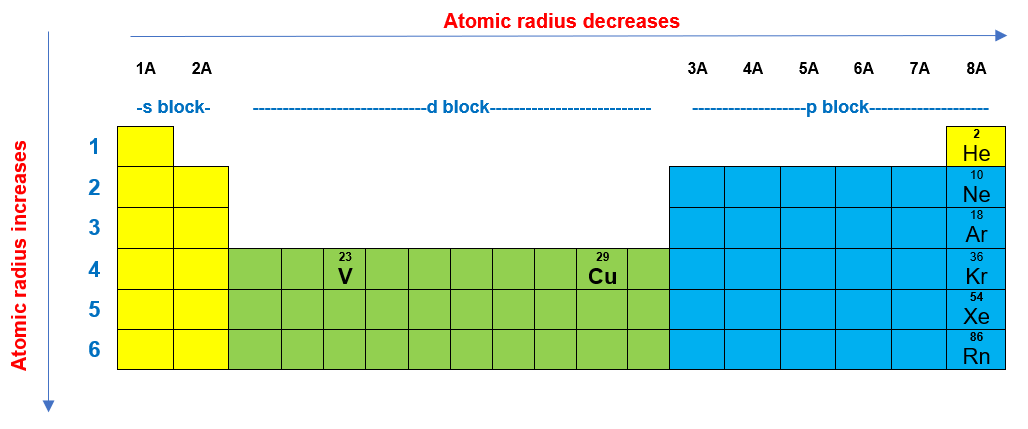Problem: Explain why vanadium (radius = 134 pm) and copper (radius = 128 pm) have nearly identical atomic radii, even though the atomic number of copper is about 25% higher than that of vanadium.

FREE Expert Solution
91% (115 ratings)
FREE Expert Solution

We have to explain why vanadium and copper have nearly the same atomic radius although the atomic number of copper is higher than that of vanadium.

The size of an atom (atomic radius) depends upon:

• The number of energy levels (shells)
• The strength of attractive forces between the nucleus and the valence electrons
• Shielding that results due to inner electrons.91% (115 ratings)
Problem Details

What scientific concept do you need to know in order to solve this problem?

Our tutors have indicated that to solve this problem you will need to apply the Periodic Trend: Atomic Radius concept. You can view video lessons to learn Periodic Trend: Atomic Radius Or if you need more Periodic Trend: Atomic Radius practice, you can also practice Periodic Trend: Atomic Radius practice problems .

How long does this problem take to solve?

Our expert Chemistry tutor, Kaitlyn took 7 minutes to solve this problem. You can follow their steps in the video explanation above.

What professor is this problem relevant for?

Based on our data, we think this problem is relevant for Professor Marin's class at UCF.

What textbook is this problem found in?

Our data indicates that this problem or a close variation was asked in . You can also practice practice problems .